Let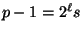,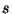odd. To find a quadratic non-residue, compute continuously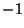,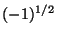,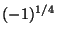,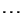,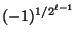. One can quickly computefrom an algorithm due to Schoof, but one gets stuck at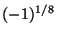.

The idea: in primality testing, we want to know if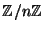is a field, so we embed it into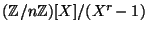; this ring has enough structure to pull out a nice algorithm. Assume that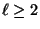, and we try only to compute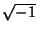. Now we embed in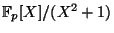. Consider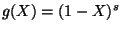. Observe that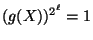in, so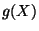is a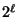th root of unity.

Assume that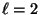. In this case,is a fourth root of unity. But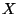is also a fourth root, so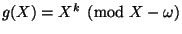where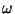is the real' fourth root of unity. Consider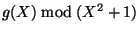: observe that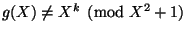for any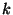. For suppose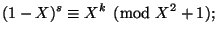then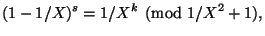so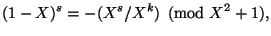hence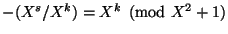, a contradiction becauseis odd. So compute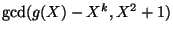, for each, one of them will factor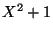.

If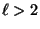, then you cannot argue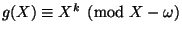. If, then it is possible thatis an eighth root or a sixteenth root or so on. Suppose thatmodulois an eighth root, for example. Then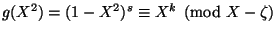for some factor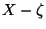of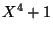andodd. But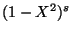is even, so it cannot be an odd power, so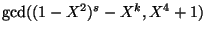will give either a linear factor (in which case we are done) or a product of quadratic factors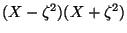or the products similar to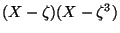and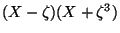.

Let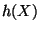be a quadratic factor of. Now if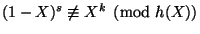, thencan be factored and we are done. Suppose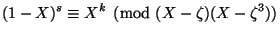and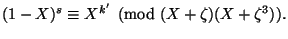If you replaceby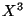, then you get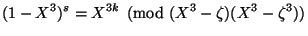so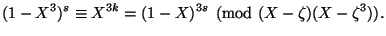That means that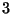is introspective for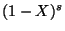. The same argument applies to the other congruence, so one obtains thatis introspective for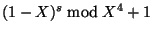.

Now try all over again now with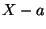replacing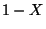; the bad case will be when we haveis introspective for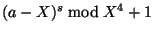for a large number of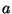. Here we get stuck, but this should be impossible.

Remarks.

1. There was a solution due to Lehmer which says for any fixed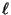that you can solve this problem? (Cohen) But that assumes the existence of a nonresidue to begin with, which is exactly our problem. (Bernstein) But also this doesn't seem to scale well. (Cohen) There is a strategy to deal with this, and we always work modulo a degree four polynomial. (Agrawal)
2. The fact that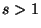means that the same techniques as in the primality test do not seem to apply. Can you solve the problem if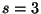, or for other small? (Lenstra) The case of a Fermat prime is trivial (is a nonresidue, and there probably aren't any above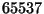). (Lenstra, Elkies) It seems as though ifis bounded, there are only a finite number of problematic. For example,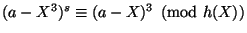does not hold for many'(Pomerance).
3. One strategy to solve this problem: translate this problem into rings and stare at it. (Lenstra)
4. Is there a strategy to deal with cases beyond eighth roots (where we have the special situation that every odd integer has square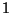)? (Elkies) Yes, but we need to solve this problem first. (Agrawal)
5. How many values ofdo you need? (Voloch) It seems unlikely you can generate the whole group with the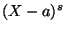without the GRH. (Bernstein)

Back to the main index for Future directions in algorithmic number theory.